## 50 Reputation

2 years, 175 days

## Fourier Series package installation prob...

Maple 2022

Dear all:

I am using maple2022 to learn Fourier Series package - Maple Application Center (maplesoft.com) . the author provide the package here:

cgi.math.muni.cz/kriz/xsrot/fourierseries/  I use the lastest version: Package FourierTrigSeries version 0.41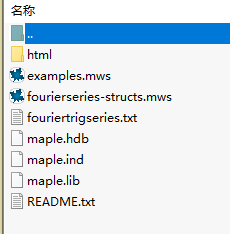there is no .mla so I do not know how to use this package.(the package is a little old).

please enlight me if you know how to install this old package.

but the author provide the code inside fourierseries-structs.mws

here is what I do:

1. first delete some lines which is not useful(which I shared in the attachment as FourierTrigSeries.mw)

and remain everything inside module and save as .mw (maybe the orginal .mws is a old version of maple files)

2. export as .FourierTrigSeries.mpl (also attached)

3. use the examples.mws (the code also from author)

I got error as: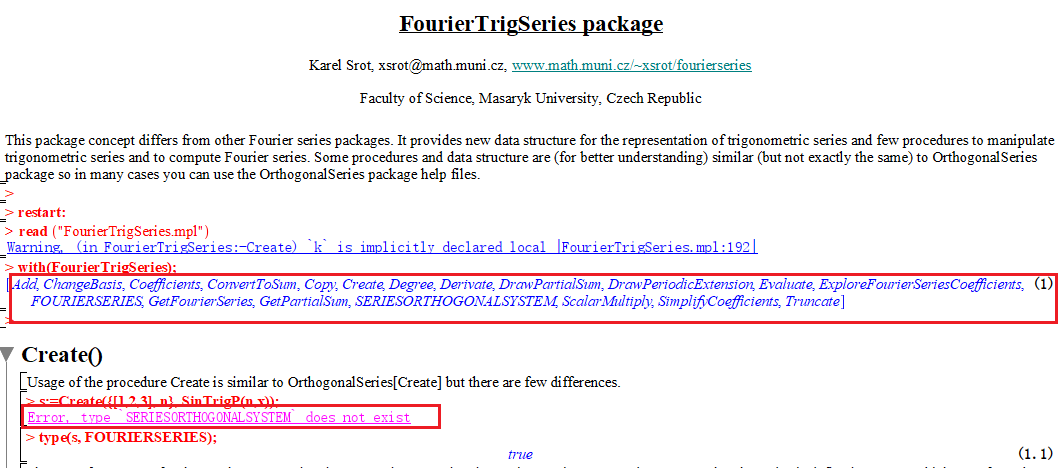the package can be recognized, but the first line wil give some error which is out of my knowledge field.

everything mentioned above is here, including the original author's files.

fouriertrigseries_0_41en_mapleprime.zip

Could you please have a look. your idea is valuable to me.

PS: this package is not available The Fourier Series package for Maple - Maple Application Center (maplesoft.com) whenI try to learn  Teaching Fourier Series with Maple II

if anyone keep it before, please share it.

rockyicer

## non-Linear equations solving issues(5 un...

Maple 2022

dear all:

here I try to repeat the results as follows:

(23) is my target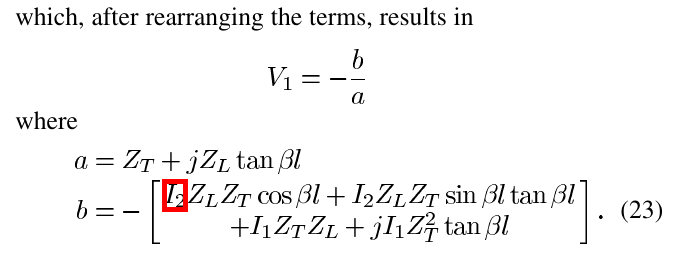my 2 solving processes are included in the attachment.

using LinearSolve(A, b) and regular solve command can not generate results as (23)

question_DHT.mw

best regards

## re-orgnize the expression by my specifie...

Maple 2022

Dear all:

I am trying to use the subs command to replace 5 varibles with other specific 5 varibles.

to be simple, here is issue.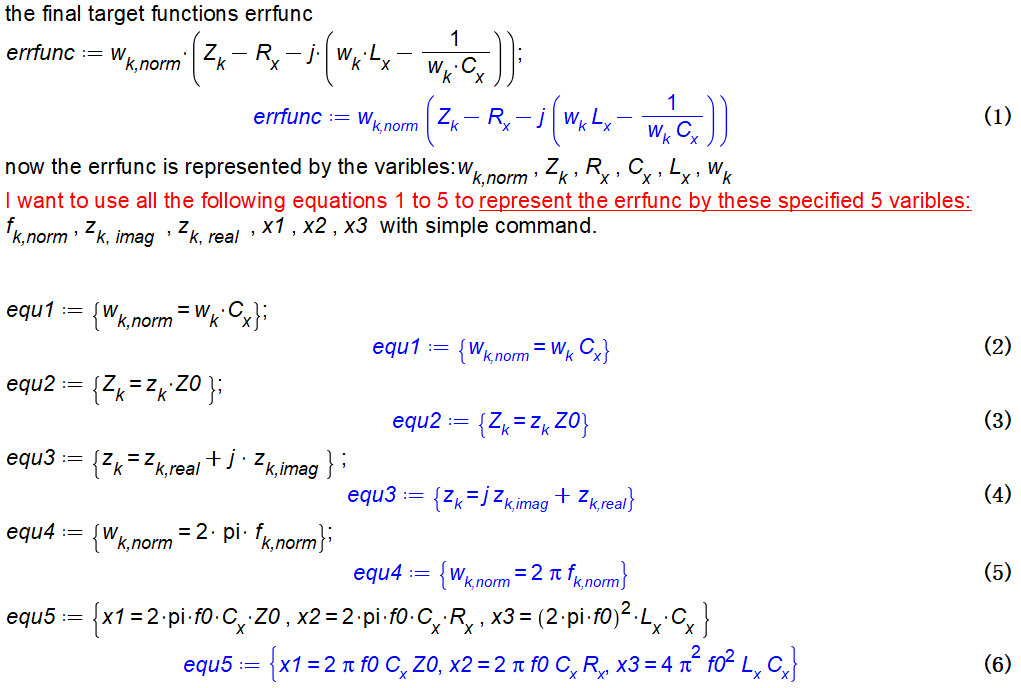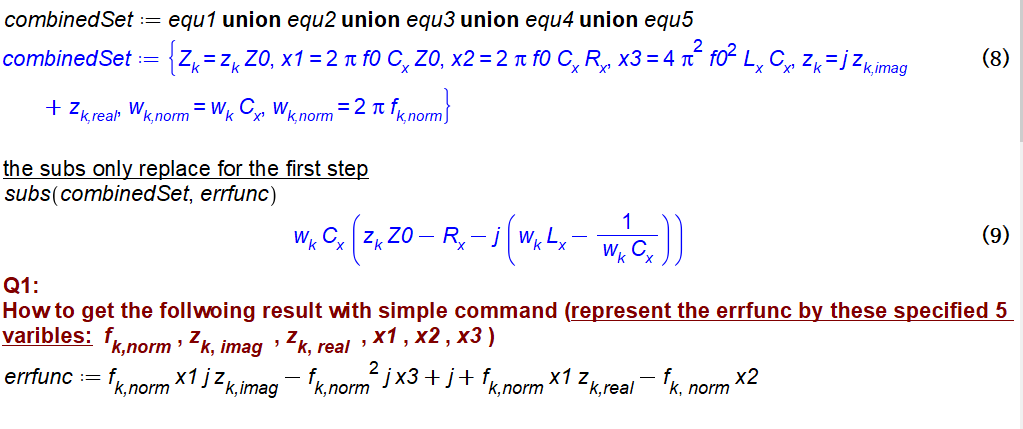Now I and using the stupid way(manually replace eachstep by my self) which did work but with some small issue such as  simplify or subs not work for replacements

all the steps I did are contained inside here:

ModelExtrinsicParasiticExtraction_QuestionsV2.mw

best regards

## solve equations issue (15equations with ...

Maple 2022

dear all,

I am trying to use the solve to get symbolic answer.

to be simple, here is issue:

I have 15equations with 16 varibles.

the 15 equation as follows:

first 4 equation set

```equList_Ya :=

[ Va1 Ya11 + Va2 Ya12 + Va3 Ya13 + Va4 Ya14 = Ia1,

Va1 Ya21 + Va2 Ya22 + Va3 Ya23 + Va4 Ya24 = Ia2,

Va1 Ya31 + Va2 Ya32 + Va3 Ya33 + Va4 Ya34 = Ia3,

Va1 Ya41 + Va2 Ya42 + Va3 Ya43 + Va4 Ya44 = Ia4]```

2nd 4 equation set

```equList_Yb :=

[ Vb1 Yb11 + Vb2 Yb12 + Vb3 Yb13 + Vb4 Yb14 = Ib1,

Vb1 Yb21 + Vb2 Yb22 + Vb3 Yb23 + Vb4 Yb24 = Ib2,

Vb1 Yb31 + Vb2 Yb32 + Vb3 Yb33 + Vb4 Yb34 = Ib3,

Vb1 Yb41 + Vb2 Yb42 + Vb3 Yb43 + Vb4 Yb44 = Ib4]```

the 3rd 4 equation set

```equList_ConnectCondition :=

[Ia3 = -Ib1, Ia4 = -Ib2, Va3 = Vb1,  Va4 = Vb2]```

the 4th 3 equation set

` equList_Y11Condition := [Va2 = 0, Vb3 = 0, Vb4 = 0]`

so total equation number is 4+4+4+3=15

```equList_Y11all := {Ia3 = -Ib1, Ia4 = -Ib2, Va2 = 0, Va3 = Vb1, Va4 = Vb2, Vb3 = 0,
Vb4 = 0, Va1*Ya11 + Va2*Ya12 + Va3*Ya13 + Va4*Ya14 = Ia1,
Va1*Ya21 + Va2*Ya22 + Va3*Ya23 + Va4*Ya24 = Ia2,
Va1*Ya31 + Va2*Ya32 + Va3*Ya33 + Va4*Ya34 = Ia3,
Va1*Ya41 + Va2*Ya42 + Va3*Ya43 + Va4*Ya44 = Ia4,
Vb1*Yb11 + Vb2*Yb12 + Vb3*Yb13 + Vb4*Yb14 = Ib1,
Vb1*Yb21 + Vb2*Yb22 + Vb3*Yb23 + Vb4*Yb24 = Ib2,
Vb1*Yb31 + Vb2*Yb32 + Vb3*Yb33 + Vb4*Yb34 = Ib3,
Vb1*Yb41 + Vb2*Yb42 + Vb3*Yb43 + Vb4*Yb44 = Ib4}```

the 16 varible is

```var_Y11 :=

[ Va1, Va2, Va3, Va4,

Ia1, Ia2, Ia3, Ia4,

Vb1, Vb2, Vb3, Vb4,

Ib1, Ib2, Ib3, Ib4]```

I hope to get Ia1=f(Va1) to calculate Y11(=Ia1/Va1)

but the solve give nothing:

`Y11_all := solve(equList_Y11all, {Ia1}, symbolic = true);                        `

Y11_all := NULL

Question1: why the above solve output nothing???

since not work,so I try another way:

```polys_Y11 := map(lhs - rhs = 0, equList_Y11all);
polys_Y11 := {Va2 = 0, Vb3 = 0, Vb4 = 0, Ia3 + Ib1 = 0,

Ia4 + Ib2 = 0, Va3 - Vb1 = 0, Va4 - Vb2 = 0,

Va1 Ya11 + Va2 Ya12 + Va3 Ya13 + Va4 Ya14 - Ia1 = 0,

Va1 Ya21 + Va2 Ya22 + Va3 Ya23 + Va4 Ya24 - Ia2 = 0,

Va1 Ya31 + Va2 Ya32 + Va3 Ya33 + Va4 Ya34 - Ia3 = 0,

Va1 Ya41 + Va2 Ya42 + Va3 Ya43 + Va4 Ya44 - Ia4 = 0,

Vb1 Yb11 + Vb2 Yb12 + Vb3 Yb13 + Vb4 Yb14 - Ib1 = 0,

Vb1 Yb21 + Vb2 Yb22 + Vb3 Yb23 + Vb4 Yb24 - Ib2 = 0,

Vb1 Yb31 + Vb2 Yb32 + Vb3 Yb33 + Vb4 Yb34 - Ib3 = 0,

Vb1 Yb41 + Vb2 Yb42 + Vb3 Yb43 + Vb4 Yb44 - Ib4 = 0}```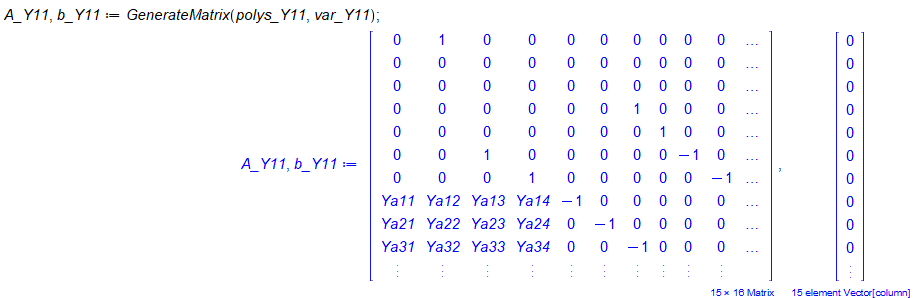and solve it with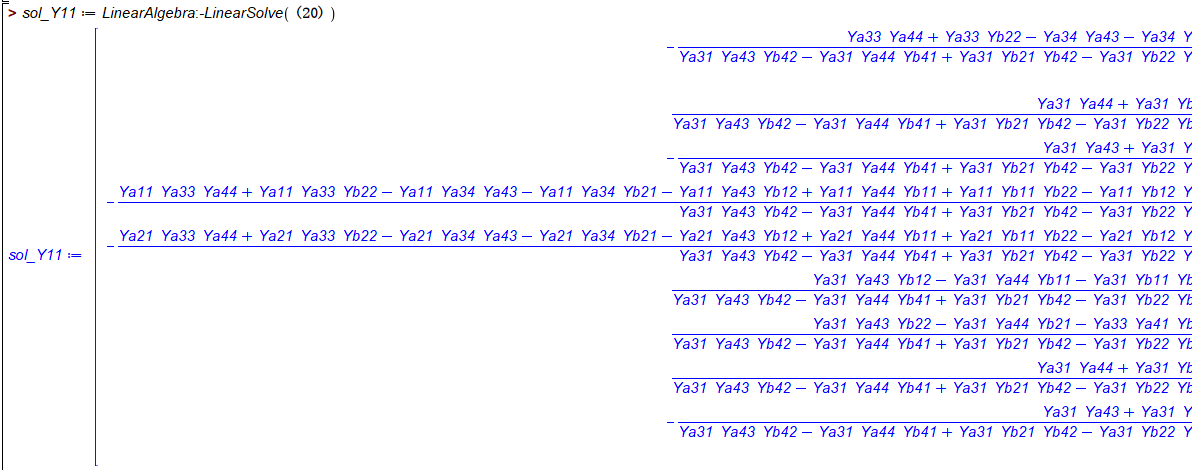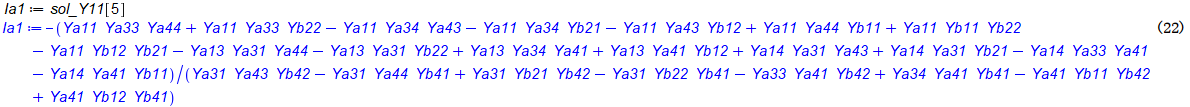what I expected is Ia1=f(Va1) which Va1 as indepentent,but the above solution have no Va1
Question2: is the (22) give the right answer for Ia1 ? what is the indepent var for the above solution?

here is my full maple worksheet

CoupleLineSeriesConnect_Question.mw